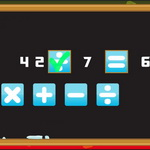Elementary Arithmetic Game

Elementary Arithmetic Game

Description

Choose the right elementary arithmetic and complete the equation! You have 60 seconds to complete a many equation as possible. In each equation, the elementary arithmetic is missing and you have to choose the right one based on the numbers and answer and complete the equation. When your 60 seconds end, your score is calculated based on the number of your correct and wrong answers. So be quick and do your best to correctly answer as many questions as you can.